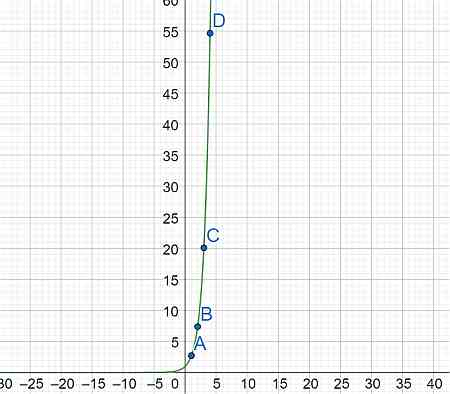# What does e^x equal?

## Question:

What does {eq}e^x {/eq} equal?

## Graphing exponentials:

The nature of exponentials is quite interesting. The {eq}'e' {/eq} in {eq}e^{x} {/eq} represents an important mathematical constant. The number {eq}e {/eq} attains a value of around {eq}2.718 {/eq}.

{eq}e^{x} {/eq} can be graphed by plotting some points in the cartesian plane as {eq}(x,e^{x}) {/eq}

Sr No. x y
1 1 e
2 2 7.389
3 3 20.085
4 4 54.598We see that the rate of increase in the function is very evident. So, this is how {eq}e^{x} {/eq} looks like.